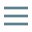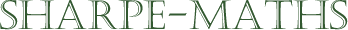Previous topic:
Primes
Current topic:
HCF (GCD) & LCM
Next topic:
Introduction to fractions

### HCF (GCD)

The Highest Common Factor (HCF) is often called the Greatest Common Divisor (GCD) in the USA and much of the rest of the world.

The HCF of a set of numbers is the biggest number which is a factor of both numbers. It is a way of examining underlying similarities in numbers, which can help us to categorise the numbers. Let us begin with a simple example:

Find the HCF of the numbers 24 and 27. This can also be written using the following notation HCF(24, 27), which is a handy shortcut.

We begin by using prime factor decomposition on both numbers. $27 = 3^3$ and $24 = 2^3 \times 3$Now draw a Venn diagram with 2 interlocking circles. Then arrange the numbers in the circles, beginning at the centre. $3$ is the only prime common to both so 3 goes in the middle and the rest of the primes are arranged in the other sections. The HCF is the product of any number(s) in the intersection. In this case, HCF(24, 27) = 3.

### LCM

The Lowest Common Multiple (LCM), also called the Least Common Multiple, is the smallest number which is a multiple of the numbers in the problem. It is worth noting here, that the LCM is generally bigger than the HCF. The HCF is always less than or equal to any of the numbers and the LCM is always greater than or equal to any of the numbers.

The LCM of a pair of numbers can be found as a by-product of finding the HCF. Let us return to the previous example, using the numbers 24 and 27.

Examine the numbers inside the Venn diagram and write them down as a product. $2^3 \times 3^3 = 216$.

It is worth considering here that there is a connection between the LCM, HCF and product of the numbers. Each of the following formulae are rearrangements of the others. In this instance, we are comparing the LCM, HCF & product of the two numbers $p$ and $q$.

$LCM(p, q) = \frac{pq}{HCF(p, q)}$.

$LCM(p, q) \times HCF(p, q) = pq$.

$HCF(p, q) = \frac{pq}{LCM(p, q)}$.